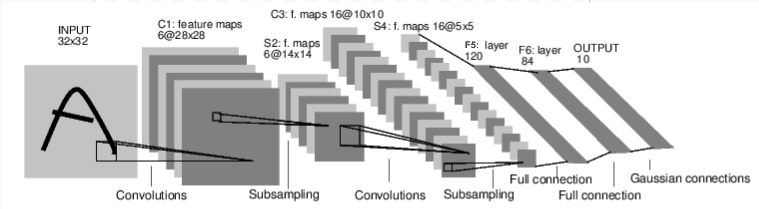pytorch入门笔记-03-神经网络## 前言1. 定义包含一些可学习的参数(或者叫权重)神经网络模型；
2. 在数据集上迭代；
3. 通过神经网络处理输入；
4. 计算损失(输出结果和正确值的差值大小)；
5. 将梯度反向传播回网络的参数；
6. 更新网络的参数，主要使用如下简单的更新原则：weight = weight - learning_rate * gradient

## 定义网络

import torch
import torch.nn as nn
import torch.nn.functional as F

class Net(nn.Module):
def __init__(self,):
super(Net, self).__init__()
# 输入图片通道数为 1，输出通道数为 6，卷积核大小为 (5, 5)
self.conv1 = nn.Conv2d(1, 6, 5)
# 输入图片通道数为 6，输出通道数为 16，卷积核大小为 (5, 5)
self.conv2 = nn.Conv2d(6, 16, 5)
self.fc1 = nn.Linear(16 * 5 * 5, 120)
self.fc2 = nn.Linear(120, 84)
self.fc3 = nn.Linear(84, 10)

def forward(self, x):
# 最大池化层，池化层窗口大小为 (2, 2)
x = F.max_pool2d(F.relu(self.conv1(x)), 2)
x = F.max_pool2d(F.relu(self.conv2(x)), 2)
# 改变数据的维度
x = x.view(-1, self.num_flat_features(x))
x = F.relu(self.fc1(x))
x = F.relu(self.fc2(x))
x = self.fc3(x)
return x

def num_flat_features(self, x):
size = x.size()[1:]
num_features = 1
for s in size:
num_features *= s
return num_features

net = Net()
print(net)

Net(
(conv1): Conv2d(1, 6, kernel_size=(5, 5), stride=(1, 1))
(conv2): Conv2d(6, 16, kernel_size=(5, 5), stride=(1, 1))
(fc1): Linear(in_features=400, out_features=120, bias=True)
(fc2): Linear(in_features=120, out_features=84, bias=True)
(fc3): Linear(in_features=84, out_features=10, bias=True)
)


net.parameters() 返回可被学习的参数（权重）列表和值

params = list(net.parameters())
print(len(params))
print(params.size())  # conv1 的权重

10
torch.Size([6, 1, 5, 5])


input = torch.randn(1, 1, 32, 32)
out = net(input)
print(out)

tensor([[ 0.0172,  0.1005, -0.1940, -0.0691, -0.0525, -0.0239, -0.0056, -0.0597,


net.zero_grad()
out.backward(torch.randn(1, 10))


### note

torch.nn 只支持小批量输入。整个 torch.nn 包都只支持小批量样本，而不支持单个样本。

• torch.Tensor：一个用过自动调用 backward() 实现支持自动梯度计算的多维数组 ，并且保存关于这个向量的梯度 w.r.t.
• nn.Module：神经网络模块。封装参数、移动到 GPU 上运行、导出、加载等。
• nn.Parameter：一种变量，当把它赋值给一个 Module 时，被自动 地注册为一个参数。
• autograd.Function：实现一个自动求导操作的前向和反向定义，每个变量操作至少创建一个函数节点，每一个 Tensor 的操作都会创建一个接到创建 Tensor编码其历史 的函数的 Function 节点。

• 定义一个网络
• 处理输入，调用 backword

• 计算损失
• 更新网络权重

## 损失函数

nn 包中有很多不同的损失函数
nn.MSELoss 是一个比较简单的损失函数，它计算输出和目标间的均方误差

output = net(input)
target = torch.rand(10)
target = target.view(1, -1)
criterion = nn.MSELoss()

loss = criterion(output, target)
print(loss)

tensor(0.4526, grad_fn=<MseLossBackward0>)


input -> conv2d -> relu -> maxpool2d -> conv2d -> relu -> maxpool2d
-> view -> linear -> relu -> linear -> relu -> linear
-> MSELoss
-> loss

print(loss.grad_fn) # MSELoss

<MseLossBackward0 object at 0x7f417c5f3d30>


## 反向传播

net.zero_grad()

loss.backward()


conv1.bias.grad before backward
tensor([0., 0., 0., 0., 0., 0.])
tensor([ 0.0040,  0.0098,  0.0213, -0.0162,  0.0075, -0.0018])


nn 包，包含了各种用来构成深度神经网络构建块的模块和损失函数，完整的文档请查看 here

• 新网络的权重

## 更新权重

weight = weight - learning_rate * gradient

learning_rate = 0.01
for f in net.parameters():


import torch.optim as optim

# 创建优化器
optimizer = optim.SGD(net.parameters(), lr=0.01)

# 迭代训练